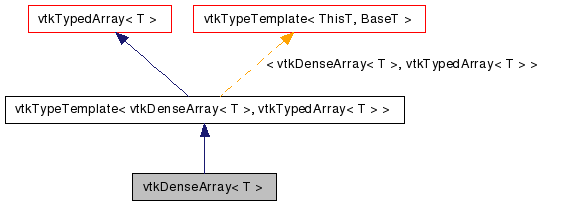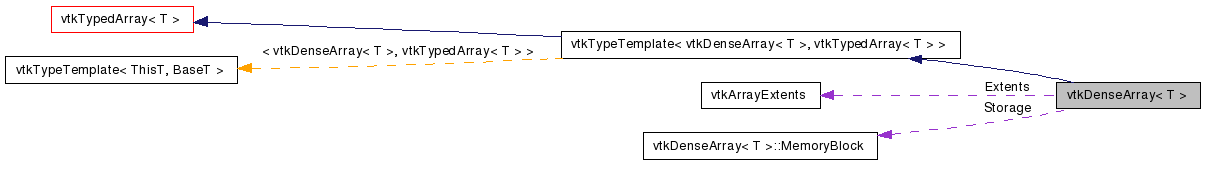# vtkDenseArray< T > Class Template Reference

`#include <vtkDenseArray.h>`

Inheritance diagram for vtkDenseArray< T >:[legend]
Collaboration diagram for vtkDenseArray< T >:[legend]

## Detailed Description

### template<typename T> class vtkDenseArray< T >

Contiguous storage for N-way arrays.

vtkDenseArray is a concrete vtkArray implementation that stores values using a contiguous block of memory. Values are stored with fortran ordering, meaning that if you iterated over the memory block, the left-most coordinates would vary the fastest.

In addition to the retrieval and update methods provided by vtkTypedArray, vtkDenseArray provides methods to:

Fill the entire array with a specific value.

Retrieve a pointer to the storage memory block.

vtkArray, vtkTypedArray, vtkSparseArray
Thanks:
Examples:
vtkDenseArray (Examples)
Tests:
vtkDenseArray (Tests)

Definition at line 60 of file vtkDenseArray.h.

void ExternalStorage (const vtkArrayExtents &extents, MemoryBlock *storage)
void Fill (const T &value)
T & operator[] (const vtkArrayCoordinates &coordinates)
const T * GetStorage () const
T * GetStorage ()
vtkDenseArray ()
~vtkDenseArray ()

## Public Types

typedef vtkArray::CoordinateT CoordinateT
typedef vtkArray::DimensionT DimensionT
typedef vtkArray::SizeT SizeT

## Public Member Functions

void PrintSelf (ostream &os, vtkIndent indent)
bool IsDense ()
const vtkArrayExtentsGetExtents ()
SizeT GetNonNullSize ()
void GetCoordinatesN (const SizeT n, vtkArrayCoordinates &coordinates)
vtkArrayDeepCopy ()
const T & GetValue (CoordinateT i)
const T & GetValue (CoordinateT i, CoordinateT j)
const T & GetValue (CoordinateT i, CoordinateT j, CoordinateT k)
const T & GetValue (const vtkArrayCoordinates &coordinates)
const T & GetValueN (const SizeT n)
void SetValue (CoordinateT i, const T &value)
void SetValue (CoordinateT i, CoordinateT j, const T &value)
void SetValue (CoordinateT i, CoordinateT j, CoordinateT k, const T &value)
void SetValue (const vtkArrayCoordinates &coordinates, const T &value)
void SetValueN (const SizeT n, const T &value)

## Static Public Member Functions

static vtkDenseArray< T > * New ()

## Classes

class  HeapMemoryBlock
class  MemoryBlock
class  StaticMemoryBlock

## Member Typedef Documentation

template<typename T>
 typedef vtkArray::CoordinateT vtkDenseArray< T >::CoordinateT

Reimplemented from vtkTypedArray< T >.

Definition at line 67 of file vtkDenseArray.h.

template<typename T>
 typedef vtkArray::DimensionT vtkDenseArray< T >::DimensionT

Reimplemented from vtkArray.

Definition at line 68 of file vtkDenseArray.h.

template<typename T>
 typedef vtkArray::SizeT vtkDenseArray< T >::SizeT

Reimplemented from vtkTypedArray< T >.

Definition at line 69 of file vtkDenseArray.h.

## Constructor & Destructor Documentation

template<typename T>
 vtkDenseArray< T >::vtkDenseArray ( ) ` [protected]`

Stores the current array extents (its size along each dimension)

template<typename T>
 vtkDenseArray< T >::~vtkDenseArray ( ) ` [protected]`

Stores the current array extents (its size along each dimension)

## Member Function Documentation

template<typename T>
 static vtkDenseArray* vtkDenseArray< T >::New ( ) ` [static]`

Create an object with Debug turned off, modified time initialized to zero, and reference counting on.

Reimplemented from vtkObject.

template<typename T>
 void vtkDenseArray< T >::PrintSelf ( ostream & os, vtkIndent indent ) ` [virtual]`

Methods invoked by print to print information about the object including superclasses. Typically not called by the user (use Print() instead) but used in the hierarchical print process to combine the output of several classes.

Reimplemented from vtkTypedArray< T >.

template<typename T>
 bool vtkDenseArray< T >::IsDense ( ) ` [virtual]`

Returns true iff the underlying array storage is "dense", i.e. that GetSize() and GetNonNullSize() will always return the same value. If not, the array is "sparse".

Implements vtkArray.

template<typename T>
 const vtkArrayExtents& vtkDenseArray< T >::GetExtents ( ) ` [virtual]`

Returns the extent (valid coordinate range) along the given dimension.

Implements vtkArray.

template<typename T>
 SizeT vtkDenseArray< T >::GetNonNullSize ( ) ` [virtual]`

Returns the number of non-null values stored in the array. Note that this value will equal GetSize() for dense arrays, and will be less-than-or-equal to GetSize() for sparse arrays.

Implements vtkArray.

template<typename T>
 void vtkDenseArray< T >::GetCoordinatesN ( const SizeT n, vtkArrayCoordinates & coordinates )

template<typename T>
 vtkArray* vtkDenseArray< T >::DeepCopy ( ) ` [virtual]`

Returns a new array that is a deep copy of this array.

Implements vtkArray.

template<typename T>
 const T& vtkDenseArray< T >::GetValue ( CoordinateT i ) ` [virtual]`

Returns the value stored in the array at the given coordinates. Note that the number of dimensions in the supplied coordinates must match the number of dimensions in the array.

Implements vtkTypedArray< T >.

template<typename T>
 const T& vtkDenseArray< T >::GetValue ( CoordinateT i, CoordinateT j ) ` [virtual]`

Returns the value stored in the array at the given coordinates. Note that the number of dimensions in the supplied coordinates must match the number of dimensions in the array.

Implements vtkTypedArray< T >.

template<typename T>
 const T& vtkDenseArray< T >::GetValue ( CoordinateT i, CoordinateT j, CoordinateT k ) ` [virtual]`

Returns the value stored in the array at the given coordinates. Note that the number of dimensions in the supplied coordinates must match the number of dimensions in the array.

Implements vtkTypedArray< T >.

template<typename T>
 const T& vtkDenseArray< T >::GetValue ( const vtkArrayCoordinates & coordinates ) ` [virtual]`

Returns the value stored in the array at the given coordinates. Note that the number of dimensions in the supplied coordinates must match the number of dimensions in the array.

Implements vtkTypedArray< T >.

template<typename T>
 const T& vtkDenseArray< T >::GetValueN ( const SizeT n ) ` [virtual]`

Returns the n-th value stored in the array, where n is in the range [0, GetNonNullSize()). This is useful for efficiently visiting every value in the array. Note that the order in which values are visited is undefined, but is guaranteed to match the order used by vtkArray::GetCoordinatesN().

Implements vtkTypedArray< T >.

template<typename T>
 void vtkDenseArray< T >::SetValue ( CoordinateT i, const T & value ) ` [virtual]`

Overwrites the value stored in the array at the given coordinates. Note that the number of dimensions in the supplied coordinates must match the number of dimensions in the array.

Implements vtkTypedArray< T >.

template<typename T>
 void vtkDenseArray< T >::SetValue ( CoordinateT i, CoordinateT j, const T & value ) ` [virtual]`

Overwrites the value stored in the array at the given coordinates. Note that the number of dimensions in the supplied coordinates must match the number of dimensions in the array.

Implements vtkTypedArray< T >.

template<typename T>
 void vtkDenseArray< T >::SetValue ( CoordinateT i, CoordinateT j, CoordinateT k, const T & value ) ` [virtual]`

Overwrites the value stored in the array at the given coordinates. Note that the number of dimensions in the supplied coordinates must match the number of dimensions in the array.

Implements vtkTypedArray< T >.

template<typename T>
 void vtkDenseArray< T >::SetValue ( const vtkArrayCoordinates & coordinates, const T & value ) ` [virtual]`

Overwrites the value stored in the array at the given coordinates. Note that the number of dimensions in the supplied coordinates must match the number of dimensions in the array.

Implements vtkTypedArray< T >.

template<typename T>
 void vtkDenseArray< T >::SetValueN ( const SizeT n, const T & value ) ` [virtual]`

Overwrites the n-th value stored in the array, where n is in the range [0, GetNonNullSize()). This is useful for efficiently visiting every value in the array. Note that the order in which values are visited is undefined, but is guaranteed to match the order used by vtkArray::GetCoordinatesN().

Implements vtkTypedArray< T >.

template<typename T>
 void vtkDenseArray< T >::ExternalStorage ( const vtkArrayExtents & extents, MemoryBlock * storage )

Initializes the array to use an externally-allocated memory block. The supplied MemoryBlock must be large enough to store extents.GetSize() values. The contents of the memory must be stored contiguously with fortran ordering, Dimension-labels are undefined after calling ExternalStorage() - you should initialize them accordingly. The array will use the supplied memory for storage until the array goes out of scope, is configured to use a different memory block by calling ExternalStorage() again, or is configured to use internally-allocated memory by calling Resize(). Note that the array will delete the supplied memory block when it is no longer in use. caller's responsibility to ensure that the memory does not go out-of-scope until the array has been destroyed or is no longer using it.

template<typename T>
 void vtkDenseArray< T >::Fill ( const T & value )

Fills every element in the array with the given value.

template<typename T>
 T& vtkDenseArray< T >::operator[] ( const vtkArrayCoordinates & coordinates )

Returns a value by-reference, which is useful for performance and code-clarity.

template<typename T>
 const T* vtkDenseArray< T >::GetStorage ( ) const

Returns a read-only reference to the underlying storage. Values are stored contiguously with fortran ordering.

template<typename T>
 T* vtkDenseArray< T >::GetStorage ( )

Returns a mutable reference to the underlying storage. Values are stored contiguously with fortran ordering. Use at your own risk!

The documentation for this class was generated from the following file:

Generated on Wed Aug 24 11:33:45 2011 for VTK by1.5.6